# The Book Stacking Problem

#### (An archive question of the week)

A recent question asked about a well-known problem about stacking books (or cards, or dominoes) so that the top one extends beyond the base, giving a link to one of many explanations of it – but one, like many, that doesn’t quite fill in all the details. Doctor Rick responded with a link to an answer he gave 5 years ago. That’s what we’ll look at today.

## The problem

There are many versions of this problem; here is the one Kalyan asked about in mid-August, which is reportedly from an edition of Stewart’s Calculus text:

Suppose you have a large supply of books, all the same size, and you stack them at the edge of a table, with each book extending farther beyond the edge of the table than the one beneath it. Show that it is possible to do this so that the top book extends entirely beyond the table.

In fact, show that the top book can extend any distance at all beyond the edge of the table if the stack is high enough.

Use the following method of stacking: The top book extends half its length beyond the second book. The second book extends a quarter of its length beyond the third. The third extends one-sixth of its length beyond the fourth, and so on. (Try it yourself with a deck of cards.) Consider centers of mass.

In real life, you can just push each book (or block, or card) gently until it’s almost ready to tip over, starting from the top. Here’s what I got when I did that with some actual books: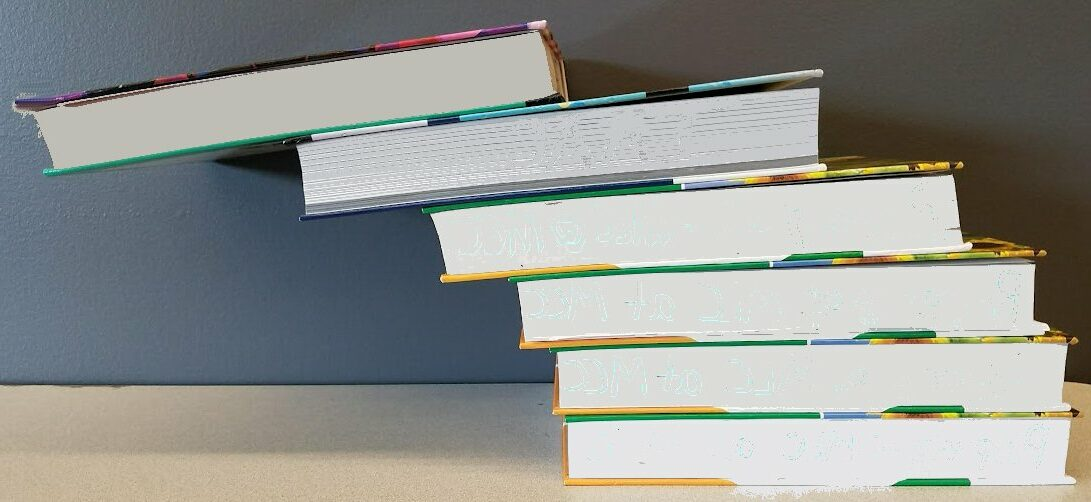It doesn’t really work as well as the theory!

It works a little better with dominoes, which are more rigid: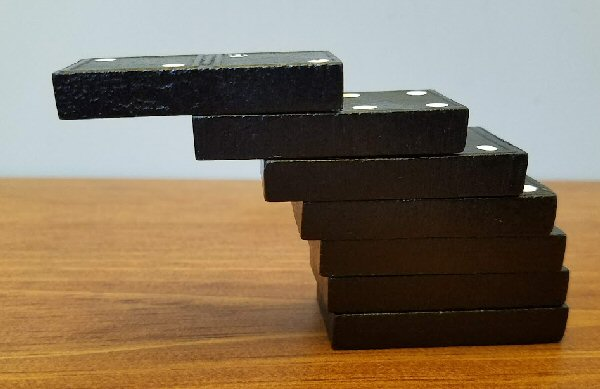Our goal here is to prove that this can (in theory, though not in reality) be extended infinitely far.

The question we’ll look at, from 2017, was very similar to Kalyan’s question:

Card Stacking Problem, Redux

I'm unable to follow what is happening here:

Solution to "Stacking Dominoes"

Specifically, this passage:

For any n, the sum of the torques of the
first n blocks has to be 0. Writing this
out gives, without much difficulty, that
if the i-th block is placed at x_i, then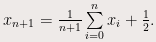How did the author get the equation? How do we make that jump "without much difficulty"? If x_(n-1) is the last displacement, then why get all of that instead of just a sum of all the displacements?

I understand the derivation immediately prior, a normal integration process; but I'm confused whether the author applies the same principle to take the next step. (When I tried to do that, I did not get the right answer.)

Maybe the author is making up a whole new thing. But if I cannot grasp the next step conceptually, how can I grasp it mathematically?

I find the indexing notation confusing, too. Wouldn't using n itself make more sense than i?

The text leading up to the quoted paragraph says,

Given n dominoes stacked following this construction, we can take the whole stack and place it on top of one more brick without displacement, and it still won’t collapse. Shifting the whole stack of n on top of the new one, at a certain point it will topple over. We want to compute the location of this point, which is exactly the point at the bottom of the nth domino around which the torque is 0. For the first domino this is 1/2, for the second 1/4, and after that you need pen and paper to proceed.

The torque of a block that is horizontally placed between the x-coordinates x0 and x0 + 1 is independent of the vertical position and is proportional to $$\int_{x_0}^{x_0+1}(x-a)dx=x_0-a+\frac{1}{2}.$$

This explanation relies heavily on physics and some calculus; but Nikola’s question is not about this part (which we’ll restate more simply below), but about the recursive formula including a summation. That’s the part we need to dig into.

### Other explanations that miss the mark

Doctor Rick replied, first looking for references he might provide:

Hi, Nikola,

Thanks for writing to Ask Dr. Math.

That explanation does look rather hard to follow. In fact, even if you get past the part you asked about, it goes on to say, "From this one can compute (this is not entirely straightforward, but not too difficult either), ..."

The author punts, admitting that the next part isn't easy ... before skipping over it anyway!

Maybe it will be more helpful if we find a better explanation of the problem and solution.

Here is a page on a respected site that explains the problem nicely with pictures:

Book Stacking Problem. From MathWorld--A Wolfram Web Resource

But it skips over most of the solution process with the words "it turns out that ..."

The reader is, of course, expected to figure that out! That’s typical of that site, which is not a tutorial. Can we find a fuller explanation?

The following document goes very deeply into the problem, discussing the physics in detail ... before generalizing the problem to such a degree that it gets VERY complex:

Overhang, by Mike Paterson and Uri Zwick

In the archives of our own service, we do have one related conversation, which falls in between these two levels of explanation:

Card Stacking Problem

I am having a hard time locating anything that gives a clear, thorough, simple explanation ...

... so I guess I'll have to write up something myself!

### Starting fresh

The question is: "How far can a stack of n blocks protrude over the edge of a table without the stack falling over?"

Rather than discussing torques, I will work with this idea: The stack of blocks (or books, or dominoes) will be stable if, for any number n of blocks counting from the top, the center of mass of that set of blocks is located over the block (or table) on which it rests. For maximum overhang, the center of mass will be directly over the *edge* of the next block down, or for the bottom block, the edge of the table.

Each block is uniform and symmetrical, so its center of mass is at the center of the block.

Here is our ideal block, with X marking its center of mass (CM):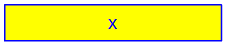Let's do as your source does, and take the width of the block to be 1 unit; and refer to the illustrations on the MathWorld page.

Here is the MathWorld picture: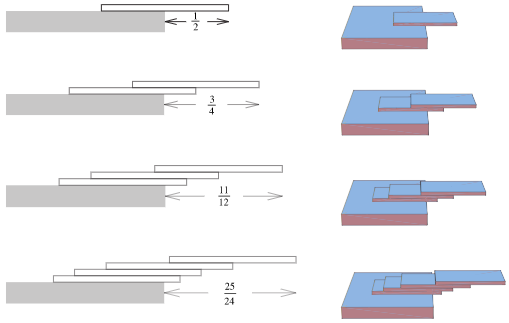A single block can protrude beyond the table by a distance 1/2, as shown in the top figure. That's because its center of mass is a distance 1/2 from the right edge of the block.

Here is that one block on the edge of the table: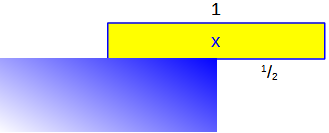At this exact location, it would be teetering on the edge, ready to fall off if a breeze pushed it a little. And if the corner were a little rounded, it would be beyond its actual support and would fall. We’re assuming mathematically perfect blocks and table!

Now, we put another block under this one, with its edge where the table's edge was. Where is the center of mass of the combination of two blocks? The center of mass of an assemblage of objects is at the weighted average of the centers of mass of the individual blocks. Let's work that out.

Here is how we find the center of mass of two objects: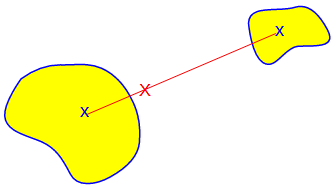The combined CM lies along the line joining the individual CMs, at a point inversely proportional to their masses, as we know from experience with see-saws: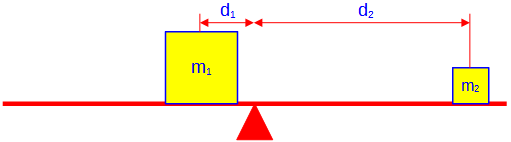Here $$\frac{d_1}{d_2}=\frac{m_2}{m_1}.$$ In other words, $$m_1d_1=m_2d_2$$. In terms of physics, the torques must be equal and opposite.

Now, suppose the two objects are located at $$x_1$$ and $$x_2$$ along a number line, and the center of mass is at $$x_c$$. Then $$m_1(x_c-x_1)=m_2(x_2-x_c)$$ Solving for $$x_c$$, $$m_1x_c-m_1x_1=m_2x_2-m_2x_c$$ $$m_1x_c+m_2x_c=m_1x_1+m_2x_2$$ $$(m_1+m_2)x_c=m_1x_1+m_2x_2$$ $$x_c=\frac{m_1x_1+m_2x_2}{m_1+m_2}.$$ This is the weighted average of the two positions.

Now we apply this to two blocks:

Measuring from the right edge of the top block, the center of mass of the top block (which I'll call x) is 1/2 unit from the right, as I said. The center of mass of the next block down is 1/2 unit to the left of its right edge, which in turn is x = 1/2 unit to the left of the right edge of the top block. This is 1/2 + 1/2 = 1 (as you can see in the second figure).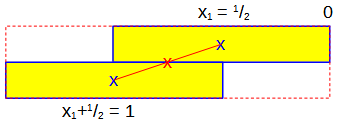Each block has the same mass, so their centers of mass are weighted equally in the weighted average. Thus, the center of mass of the top two blocks together is

x = (1/2 + 1)/2 = 3/4

Note that this is where the edge of block number 3 will be placed.

$$x_2=\frac{x_1+1}{2}=\frac{\frac{1}{2}+1}{2}=\frac{3}{4}$$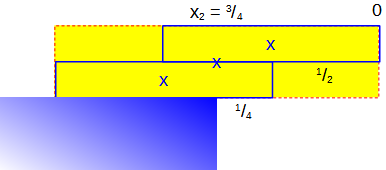We place the edge of the third block under the center of mass of the top two combined, so it will just balance: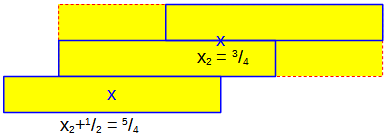Now, I'll generalize, going to x[n + 1].

Suppose we have x[n], the center of mass of the top n blocks. Block n + 1 has its right edge at x[n], and its center of mass is 1/2 unit to the left of that; that is, at x[n] + 1/2. Now we take the weighted average of this one block and the n blocks above it:

x[n + 1] = ((x[n] + 1/2) + n*x[n])/(n + 1)
= (x[n](1 + n) + 1/2)/(n + 1)
= x[n] + 1/(2(n + 1))

Here, for example, is how we find the center of mass of the first three blocks: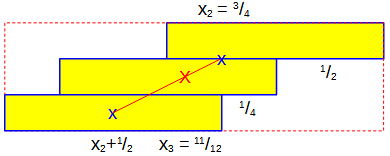$$x_3=\frac{2\left(x_2\right)+1\left(x_2+\frac{1}{2}\right)}{2+1}=\frac{\left(3x_2+\frac{1}{2}\right)}{3}=\frac{\left(3\left(\frac{3}{4}\right)+\frac{1}{2}\right)}{3}=\frac{\;\frac{11}{4}\;}{3 }=\frac{11}{12}$$

The added distance is $$\frac{1}{6}$$.

That's a recursive definition for x[n]. Working out successive values of x[n], we find

x = 1/2                          (starting point of the recursion)
x = 1/2 + 1/(2*2)
x = 1/2 + 1/4 + 1/(2*3)
x = 1/2 + 1/4 + 1/6 + 1/(2*4)

... and so on.

The successive increases (that is, the overhangs of each block) are $$\frac{1}{2}$$, $$\frac{1}{4}$$, $$\frac{1}{6}$$, $$\frac{1}{8}$$, and so on.

Thus, we see that $$x_n=\sum_{k=1}^n\frac{1}{2k}$$

Notice that we can factor 1/2 out of all the terms, leaving:

x[n] = (1/2)(1 + 1/2 + 1/3 + 1/4 + ... + 1/n)

The sum in the last set of parentheses is known as the harmonic series. You can look up more information about this series; the key point is that it diverges. That is, if you choose a great enough value for n, you can make the finite sum (nth partial sum of the infinite harmonic series) grow as large as you wish. It takes a LOT of terms, even to reach 3, but it can be done.

... *in principle*. It's worth noting that everything said here requires ideal conditions. In practice, the blocks won't be perfect; a slight rounding of the corners would be sufficient to make it impossible to reach an overhang of 3.

Here I have simulated 35 blocks using a bar chart; you can see that the offset passes 1 after 4 blocks, and surpasses 2 after 31 blocks. To reach 3, you need 227 (not shown)!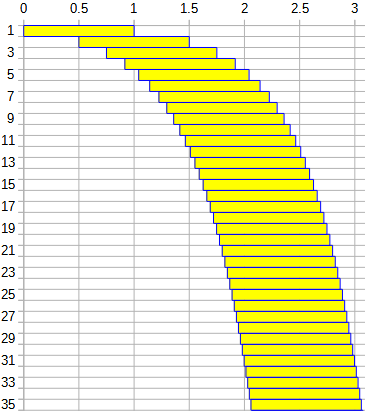This site uses Akismet to reduce spam. Learn how your comment data is processed.